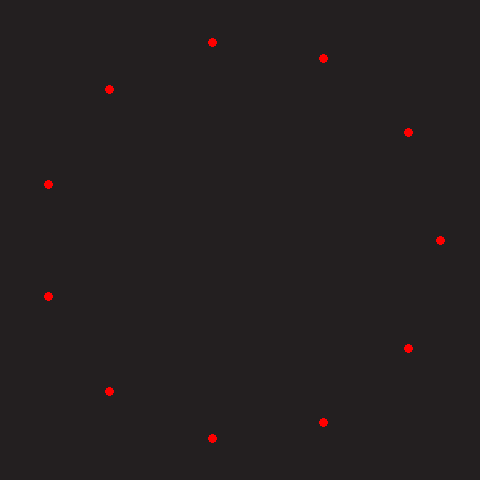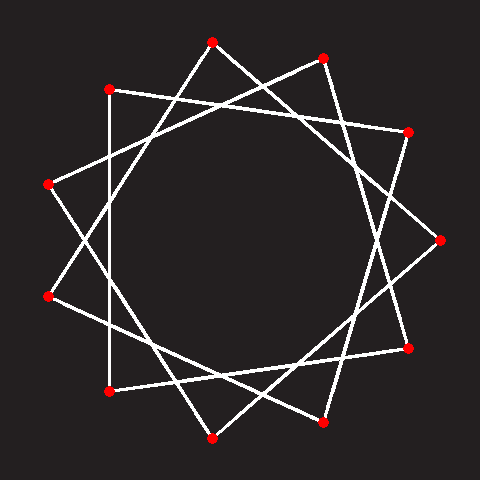# Star Polygons

(Or more formally "Self-intersecting equilateral equiangular polygons")We can also skip every second vertex to make a different type of pointed star, and again, and again … however, if we skip past more than half the vertices, we end up with duplication (symmetrical about the median number), albeit that these later stars are made from ‘winding’ the other way around.
N=11, D=1Connecting adjacent vertices makes a regular polygon.
N=11, D=2Connecting every other vertex makes the first star polygon.
N=11, D=3Connecting every third vertex makes a different star.
N=11, D=4Every fouth vertex.
N=11, D=5Every fifth vertex.
N=11, D=9Every ninth vertex.
Notice in the last example, skipping every ninth vertex produces a star that looks like the star produced by skipping every second vertex except that it appears to be constructed by winding around the opposite direction.

## Coprime

If the number of vertices, and the gap to skip are relatively prime (contain no common factors), as all our examples have been to-date, then the edges will wind around to make a star and eventually return to the starting position. You can see this in the image on the left below. However, if the there are common factors between the two numbers, this results in multiple sets of discontiguous stars; such as the image on the right.
N=11, D=3N=12, D=3If you look closely at the image on the right you can see that is a star made up of three squares.

## Naming conventions

The star shapes can be classified and described with two numbers: The number of vertices, and the number of vertices to skip. A common nomenclature for these is the Schläfli system. For example, the star polygon on the left above can be described as {11/3}, and the one on the right as {12/3}. Sometimes the common factors are taken out and you might see this as being represented as 3{4/1}.
As regards common vernacular names, they are given the suffix -gram to the Greek named number prefix. Just like we call polygons: Pentagons, Hexagons, Nonagons … a star polyon with five points is called a Pentagram, and a six pointed star polygon is caled a Hexagram
The first detailed study of these polygons was performed tbe English mathematician and cleric* Thomas Bradwardine, in the 1300's.
*He was even (briefly) The Archbishop of Canterbury, and is mentioned in Chaucer's Canterbury Tales.
Star polygons feature prominently is art, religion, and culture. For instance, {5/2} Pentagrams, and {7/2}, {7/3} Heptagrams, have been historically associated with magic and the occult. The {6/2} Hexagram is the Star of David, and the {8/2} Octagram is used by Hindu's to symbolise Ashtalakshmi.